# RD Sharma Solutions For Class 12 Maths Exercise 19.13 Chapter 19 Indefinite Integrals

RD Sharma Solutions for Class 12 Maths Exercise 19.13 Chapter 19 Indefinite Integrals is provided here.In this exercise, we shall discuss the evaluation of integrals by using trigonometric substitutions. Here, the given expression can be replaced in terms of trigonometric equations to apply standard trigonometric identities. In order to excel in the Class 12 Mathematics examinations, a structured way of understanding the concepts and solving problems is a must.

The RD Sharma Solutions is on par with these needs for a student to secure good marks in the qualification exam. Furthermore, the solutions have been created by our expert team at BYJU’S, which is of simple language with appropriate explanations, to give clarity on the concepts in the chapter. To know more about this exercise, students can refer to RD Sharma Solutions for Class 12 Maths Chapter 19 Exercise 19.13.

## Download the PDF of RD Sharma Solutions For Class 12 Chapter 19 – Indefinite Integrals Exercise 19.13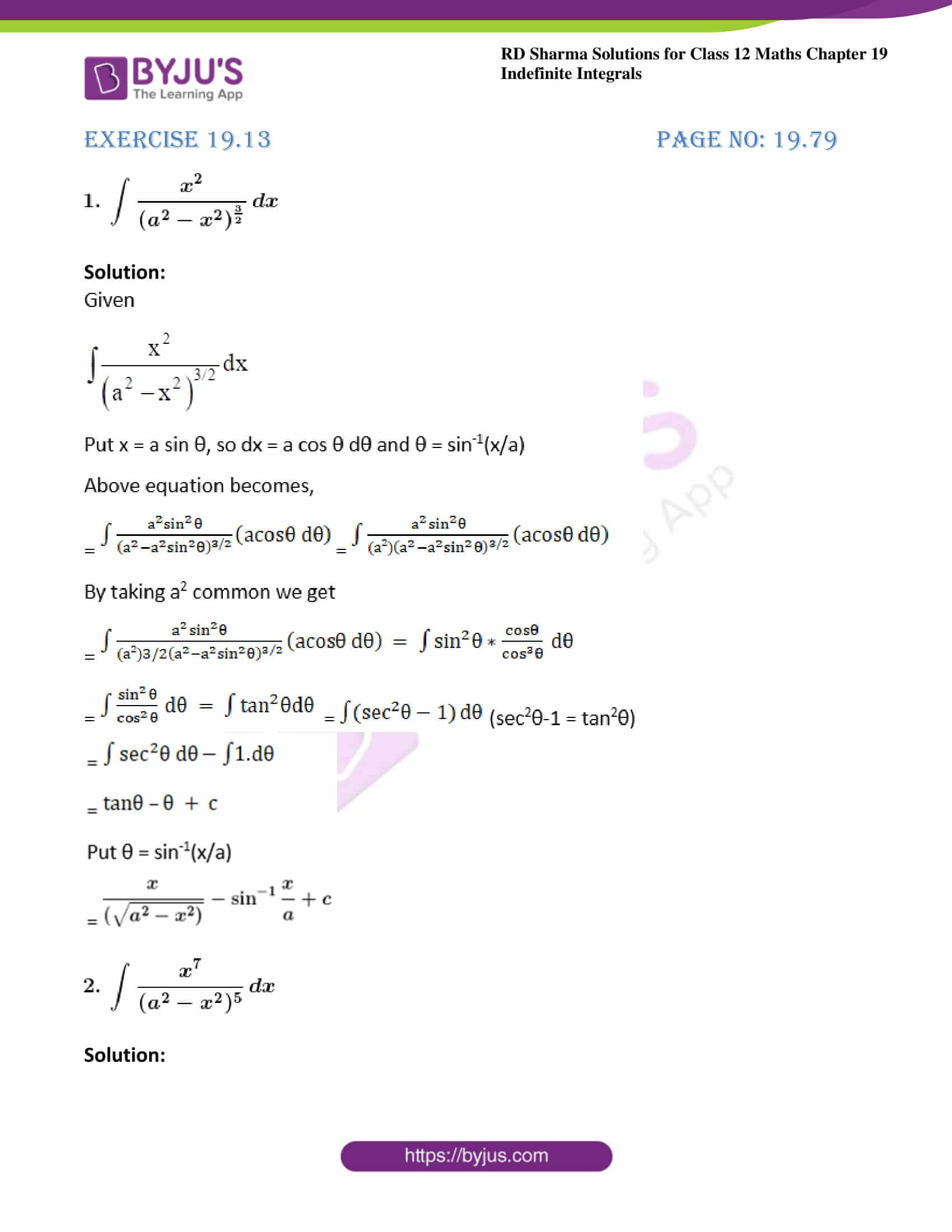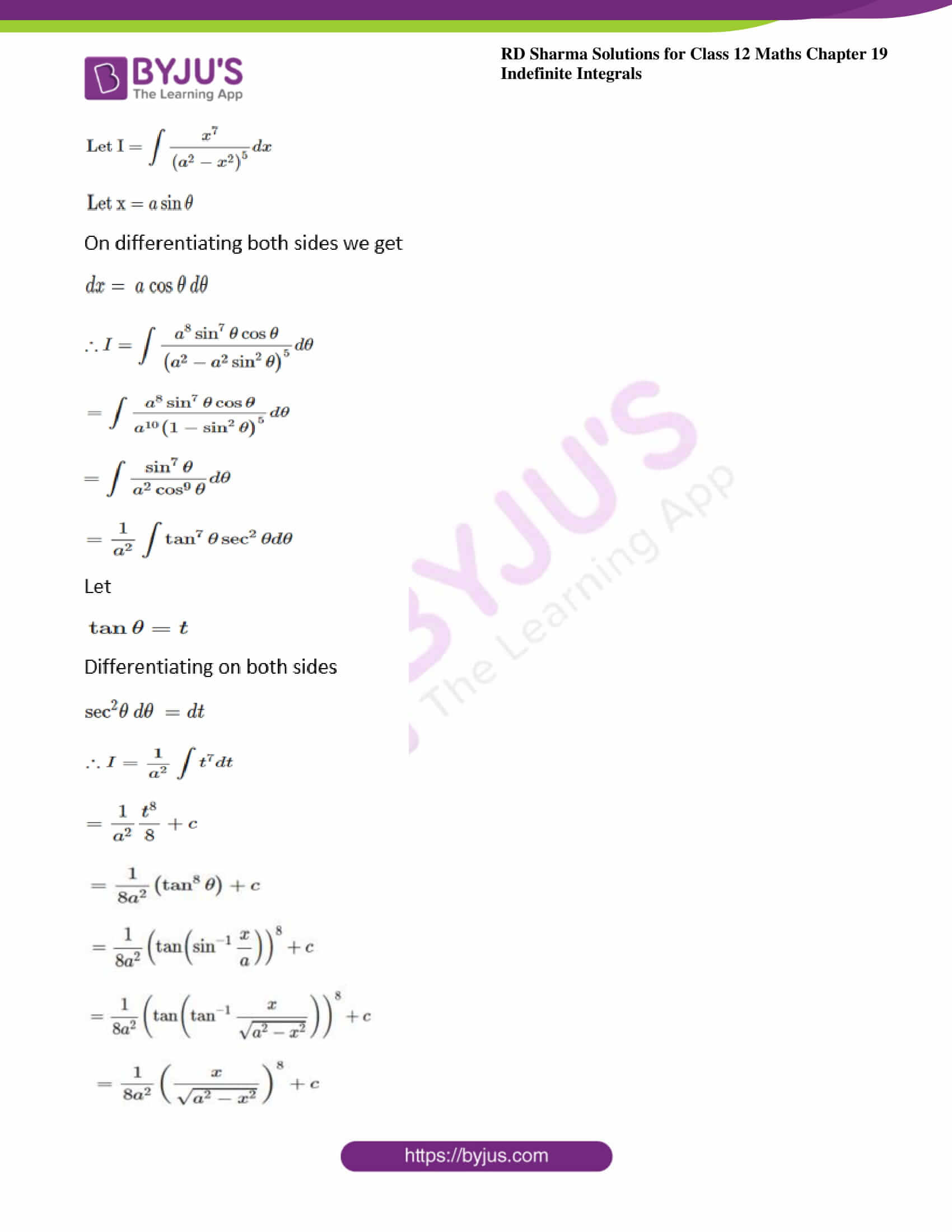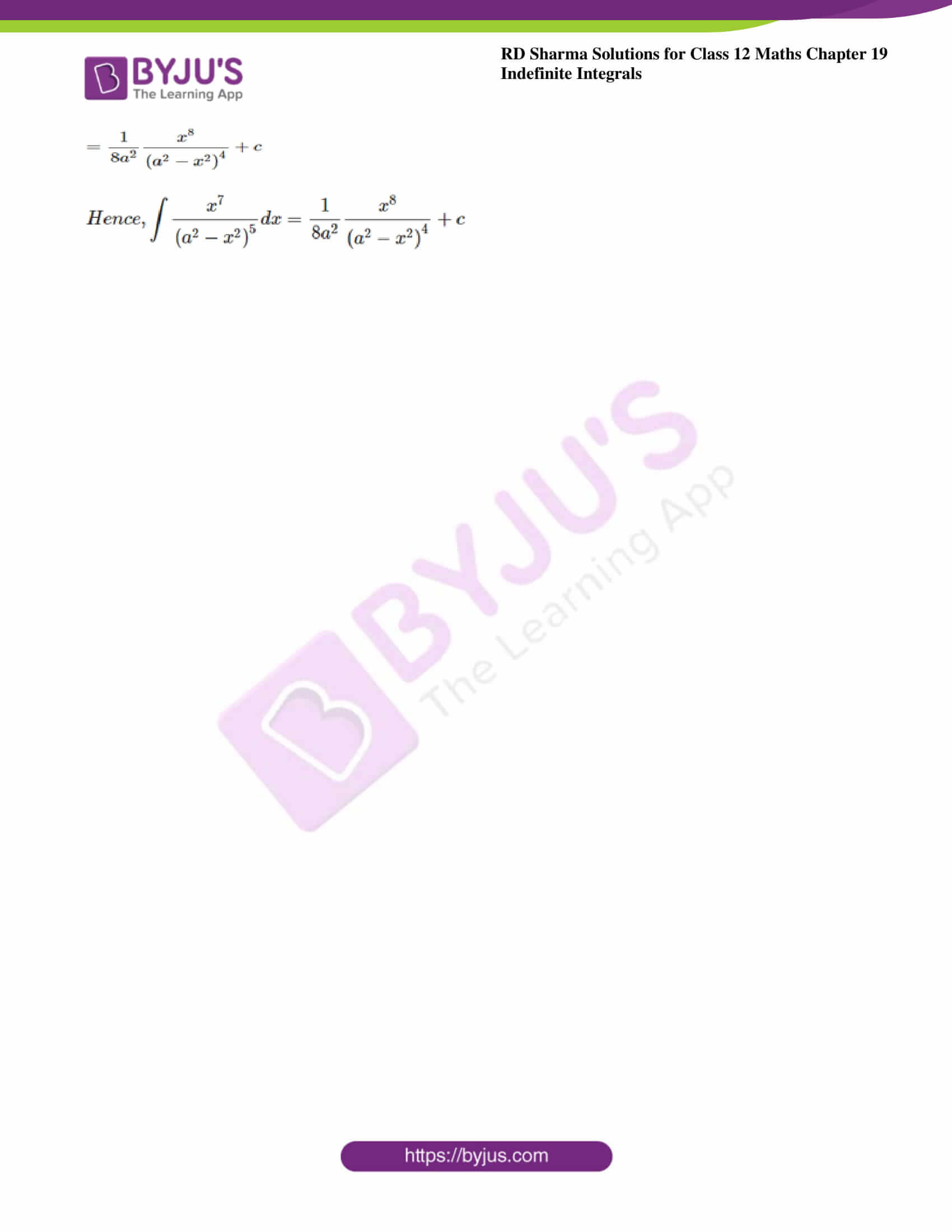### Exercise 19.13 Page No: 19.79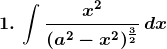Solution: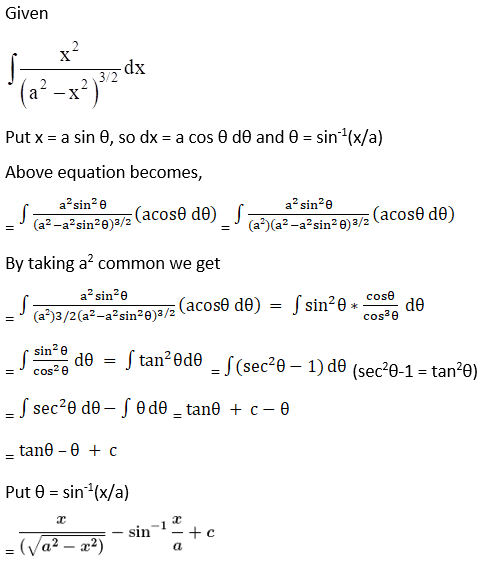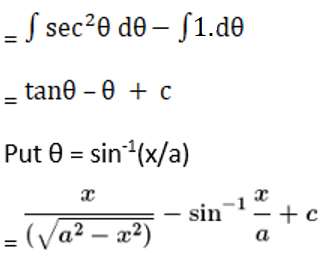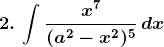Solution: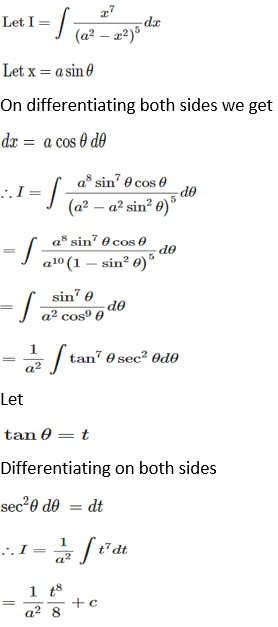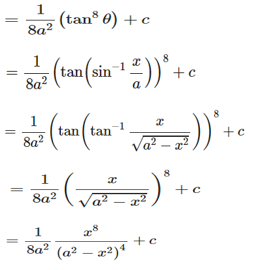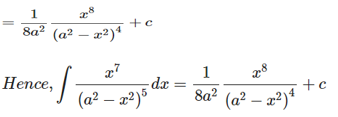### Access other exercises of RD Sharma Solutions For Class 12 Chapter 19 – Indefinite Integrals

Exercise 19.1 Solutions

Exercise 19.2 Solutions

Exercise 19.3 Solutions

Exercise 19.4 Solutions

Exercise 19.5 Solutions

Exercise 19.6 Solutions

Exercise 19.7 Solutions

Exercise 19.8 Solutions

Exercise 19.9 Solutions

Exercise 19.10 Solutions

Exercise 19.11 Solutions

Exercise 19.12 Solutions

Exercise 19.14 Solutions

Exercise 19.15 Solutions

Exercise 19.16 Solutions

Exercise 19.17 Solutions

Exercise 19.18 Solutions

Exercise 19.19 Solutions

Exercise 19.20 Solutions

Exercise 19.21 Solutions

Exercise 19.22 Solutions

Exercise 19.23 Solutions

Exercise 19.24 Solutions

Exercise 19.25 Solutions

Exercise 19.26 Solutions

Exercise 19.27 Solutions

Exercise 19.28 Solutions

Exercise 19.29 Solutions

Exercise 19.30 Solutions

Exercise 19.31 Solutions

Exercise 19.32 Solutions